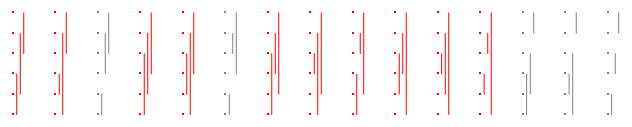Want to share your content on R-bloggers? click here if you have a blog, or here if you don't.An interesting challenge on The Riddler on the probability to see a random interval X’ing with all other random intervals when generating n intervals from Dirichlet D(1,1,1). As it happens the probability is always 2/3, whatever n>1, as shown by the R code below (where replicate cannot be replaced by rep!):

```qro=function(n,T=1e3){
quo=function(n){
xyz=t(apply(matrix(runif(2*n),n),1,sort))
sum(xyz[,1]max(xyz[,1]))<0}
mean(replicate(quo(n),T))}
```

and discussed more in details on X validated. As only a property on permutations and partitions. (The above picture is taken from this 2015 X validated post.)